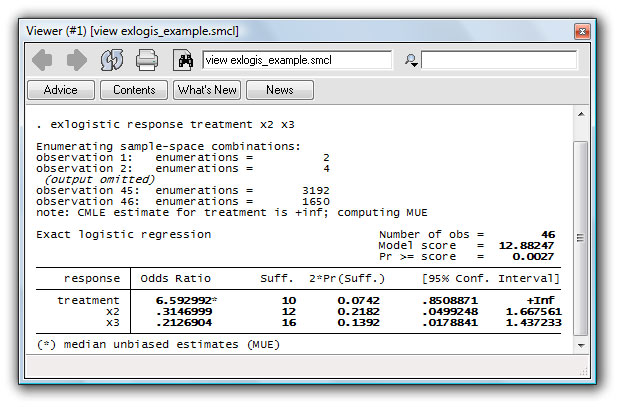»  Home »  Products »  Stata 10 »  Exact statistics

## Exact statistics

Stata 10 offers several commands for exact analysis, providing more reliable statistical inference with small-sample datasets.

• New command exlogistic fits exact logistic regression models. The dependent variable can be Bernoulli (0 or 1) or binomial (the number of successes in n trials). Exact joint hypothesis tests can be performed, and predictions with exact confidence intervals can be obtained.
• New command expoisson fits exact Poisson regression models of count outcomes. Exact joint hypothesis tests can be performed.
• Other commands for exact statistics include
Command Description
bitest Binomial probability test
cc Case–control study odds ratios
ci Centiles with confidence intervals
cs Cohort study risk differences and ratios
ir incidence-rate ratios and differences
istdize Indirect standardization
ksmirnov Two-sample Kolmogorov–Smirnov test
loneway Large one-way analysis of variance
mcc Matched case–control study statistics
median Equality-of-medians test
symmetry Symmetry and marginal homogeneity tests
tabulate2, tab2 Two-way tables with Fisher’s exact test
tetrachoric Tetrachoric correlations

### Exact logistic example

Stata’s new exact logistic regression command provides better coverage in small samples than does standard logistic regression.

It also provides parameter estimates and confidence intervals where standard asymptotic methods cannot.

Such cases include small-data problems with binary regressors for which the outcome is 1 whenever the regressor is 1.

In the example below, every treated patient exhibits a positive response. Standard logistic regression cannot estimate the treatment effect. Stata 10’s new exlogistic command can:

    . exlogistic response treatment x2 x3Parameter estimates, standard errors, and CIs are calculated on the basis of permutation without recourse to asymptotic assumptions and results.

Not only is the treatment effect estimated, but tests of significance and reported confidence intervals are based on exact methods.

Stata 10 also includes exact Poisson regression for count data.